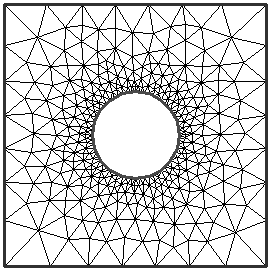The Rocscience International Conference 2021 Proceedings are now available. Read Now

## Search Results

In the Mesh Setup dialog, the default Mesh Type is Graded, which produces a good graded mesh for most models, using what is known as a quadtree nodal insertion technique. In the majority of cases you will use a Graded mesh type.

When the Mesh Type = Graded, you will be required to specify the Element Type , Gradation Factor and Number of Excavation Nodes in the Mesh Setup dialog.

In the example below:

• Number of Excavation Nodes = 60
• Element Type = Triangles## Number of Excavation Nodes

The Number of Excavation Nodes directly determines the discretization of the Excavation boundaries. If more than one Excavation boundary is defined, this number is the total for all excavations.

RS2 subdivides the Excavation boundaries in such a way that the number of excavation nodes is approximately equal to the Number of Excavation Nodes in Mesh Setup. You may notice that the actual number of Excavation nodes after discretizing may differ slightly from the Number of Excavation Nodes in the Mesh Setup option. This is normal, and will vary depending on the excavation(s).

The Number of Excavation Nodes in conjunction with the Gradation Factor, determines the discretization of all other boundaries in the model, as described below.

The Gradation Factor, in conjunction with the Number of Excavation Nodes, determines the discretization of all other boundaries in the model (e.g. External, Material, Stage, Joint).

The Gradation Factor is the ratio of:

• the average length of discretizations on Excavation boundaries, to
• the length of discretizations on the External boundary, at the maximum distance from the Excavation boundaries

The discretization of all other boundaries in the model is graded, according to the distance of the boundary from Excavation boundaries.

For a simple model where the External boundary is equally distant from the Excavation boundary, a Gradation Factor of 0.1 implies that the average length of the External boundary discretizations will be (approximately) 10 times the average length of the Excavation discretizations. This is illustrated below.

Due to the nature of the discretization process, the Gradation Factor should be considered an approximate number.

Effect of Gradation Factor on the mesh - from left to right, Gradation Factor = 0.1, 0.2, 0.3. (The Expansion Factor of the External Boundary is equal to 1 in this case).

As a rule of thumb, the farther away the External boundary is from the excavations (i.e. the greater the Expansion Factor), the smaller the Gradation Factor should be, so that you do not end up with an unnecessarily large number of elements.

## Number of External Boundary Nodes

Not all RS2 models will necessarily use Excavation boundaries. In the case of a model with no Excavation boundaries, for example:

• a slope model or surface excavation
• an Axisymmetric model
• a "joints only" model
• any other model that does not utilize an Excavation boundary

the Mesh Setup dialog will allow you to enter the Number of External Boundary Nodes, rather than the Number of Excavation Nodes. In this case, the Gradation Factor is not applicable and will be disabled, since the Gradation Factor is only relevant if Excavation boundaries exist.